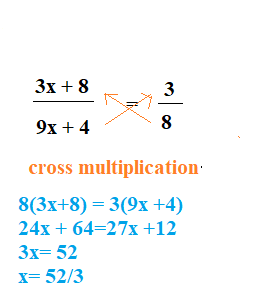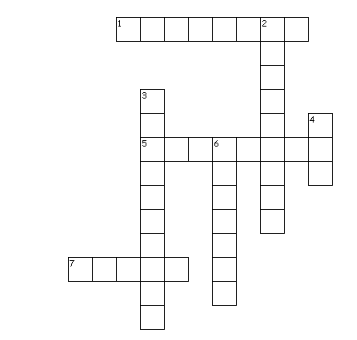# Linear equations Test Paper

In this page we have Test paper for Linear equations Class 8 maths Chapter 2 . Hope you like them and do not forget to like , social share and comment at the end of the page.
Question 1
Solve the following linear equations
1. $11 x + 10 = 10 x + 6$
2. $12 x + 5 = 5 x + 3$
3. $16 x + 9 = 9 x + 5$
4. $11 x + 2 = 2 x + 10$
5. $18 x + 4 = 4 x + 8$
6. $20 x + 10 = 10 x + 4$
7. $9 x + 6 = 6 x + 3$
8. $9 x + 3 = 3 x + 10$

These are pretty simple ,which can be obtained using transposing the values from left to right

1. $x = -4$
2. $x = - \frac{2}{7}$
3. $x = - \frac{4}{7}$
4. $x = \frac{8}{9}$
5. $x = \frac{2}{7}$
6. $x = - \frac{3}{5}$
7. $x = -1$
8. $x = \frac{7}{6}$

Question 2
Solve:
1. $\frac{3 x + 8}{9 x + 4} = \frac{3}{8}$
2. $\frac{6 x + 3}{7 x + 4} = 2$
3. $\frac{7 x + 3}{9 x + 5} = \frac{7}{3}$
4. $\frac{5 x + 4}{10 x + 10} = \frac{5}{4}$
5. $\frac{6 x + 7}{x + 6} = \frac{6}{7}$
6. $\frac{x + 4}{2 x + 4} = \frac{1}{4}$

First question is explained in below picture. We can solve the other questions similarly

1. $x = \frac{52}{3}$
2. $x = - \frac{5}{8}$
3. $x = - \frac{13}{21}$
4. $x = - \frac{17}{15}$
5. $x = - \frac{13}{36}$
6. $x = -6$

Question 3
Solve
1. $\frac{x}{8} + 6 = \frac{x}{6} + 2$
2. $\frac{x}{10} + 4 = \frac{x}{9} + 8$
3. $\frac{x}{10} + 6 = \frac{x}{3} + 8$
4. $\frac{x}{3} + 10 = \frac{x}{10} + 7$
5. $x + 5 = \frac{x}{8} + 9$
6. $\frac{x}{4} + 1 = \frac{x}{9} + 2$
7. $\frac{x}{8} + 9 = \frac{x}{10} + 6$
8. $\frac{x}{10} + 7 = \frac{x}{2} + 9$

1. $x = 96$
2. $x = -360$
3. $x = - \frac{60}{7}$
4. $x = - \frac{90}{7}$
5. $x = \frac{32}{7}$
6. $x = \frac{36}{5}$
7. $x = -120$
8. $x = -5$

Question 4
Solve each of the following equations
(a) $\frac {x+1}{4} = \frac {x-2}{2}$
(b) $1 -(p - 2) - [(p - 3) - (p - 1)] = 0$
(c) $7x - 7 = 21 -5x$
(d) $-7x = 84 +x$
(e)$0.25 (4m - 5) = 0.75m + 8$
(f) $3(x - 4) = 21$
(g)$\frac {3x}{2} - \frac {x}{3} = 8$
(h) $\frac {y}{2} -\frac {1}{4}(y- \frac {1}{3}) = \frac {1}{6} (y+1) + \frac {1}{12}$
(i) 3(2z - 3) = 12(2z + 4)

a. x=5
b. $1 -(p - 2) - [(p - 3) - (p - 1)] = 0$
$1 - p +2 - [p-3 -p+1]=0$
$1 -p +2 +2=0$
$p=5$

c. x= 7/3
d. x= -21/2
e. $0.25 (4m - 5) = 0.75m + 8$
$m -1.25=.75m + 8$
$m - .75 m = 8 + 1.25$
$.25 m= 9.25$
$m= 37$

f. x=11
g. $\frac {3x}{2} - \frac {x}{3} = 8$
$6(\frac {3x}{2} - \frac {x}{3} ) = 6 \times 8$
$9x -2x = 48$
$7x=48$
x= 48/7
h. $\frac {y}{2} -\frac {1}{4}(y- \frac {1}{3}) = \frac {1}{6} (y+1) + \frac {1}{12}$
Multiply by 12 on both sides
$6y - 3(y- \frac {1}{3}) = 2(y+1) + 1$
$6y -3y+ 1= 2y+2 +1$
$y=2$
i. $3(2z - 3) = 12(2z + 4)$
$6z -9 =24z +48$
$18z = -9-48$
$18z=-57$
$z= - \frac {57}{18}$

Question 5
The sum of three consecutive odd natural numbers is 69. Find the prime number out of these numbers

Let the numbers be x , x+2 ,x+4
Then
$x+ x+2 +x +4=69$
$3x +6=69$
$x=21$
So Numbers are 21,23,25. The prime number out of these is 23

Question 6
A positive number is 5 times another number. If 21 is added to both the numbers, then one of the new numbers become twice of another new numbers. Find the original numbers.

Let x be another number, then positive number will be 5x
Now
$5x + 21 = 2(x + 21)$
$3x= 63$
$x= 21$
So numbers are 105 , 21

Question 7
The shifting of a number from one side of an equation to other is called
(a) Transposition
(b) Distributivity
(c) Commutativity
(d) Associativity

(a)

Question 8
Crossword PuzzleAcross
1. Shifting of variable or constant from one side to another side
5. A statement formed when an equal sign in placed between two expressions.
7. The value of x for 2x -4=10
Down
2. The values for which the equation LHS equals RHS
3. If a term of an expression consists of a number multiplied by one or more variables, this number is the __________________ of the term
4. highest power of the variable in linear equation
6. Branch of mathematics concerned with operation by symbolic numbers

1. tranpose
2. solutions
3. Coefficent
4. one
5. equation
6. Algebra
7. seven

## Summary

This Test Paper for Linear equations Class 8 maths Chapter 2 is prepared keeping in mind the latest syllabus of CBSE . This has been designed in a way to improve the academic performance of the students. If you find mistakes , please do provide the feebback on the mail.